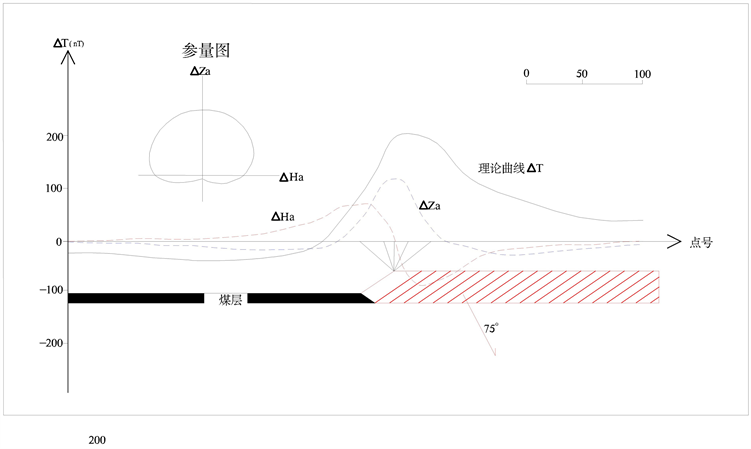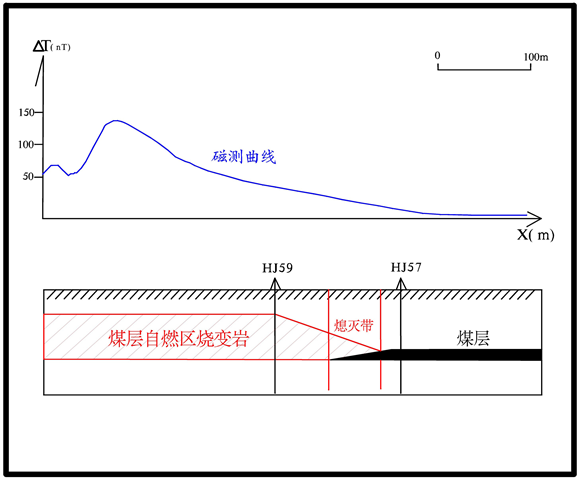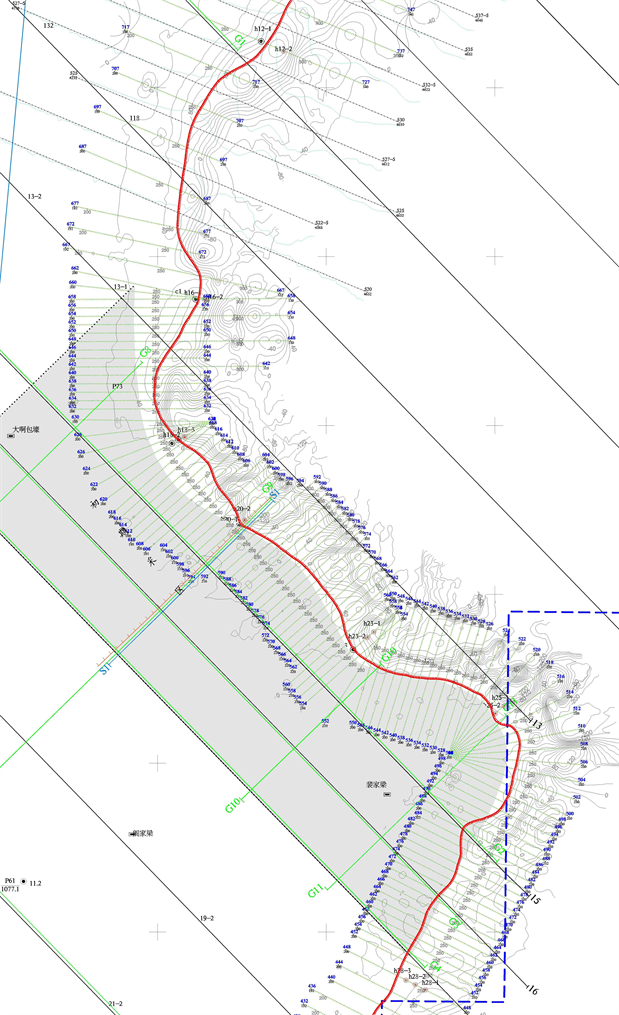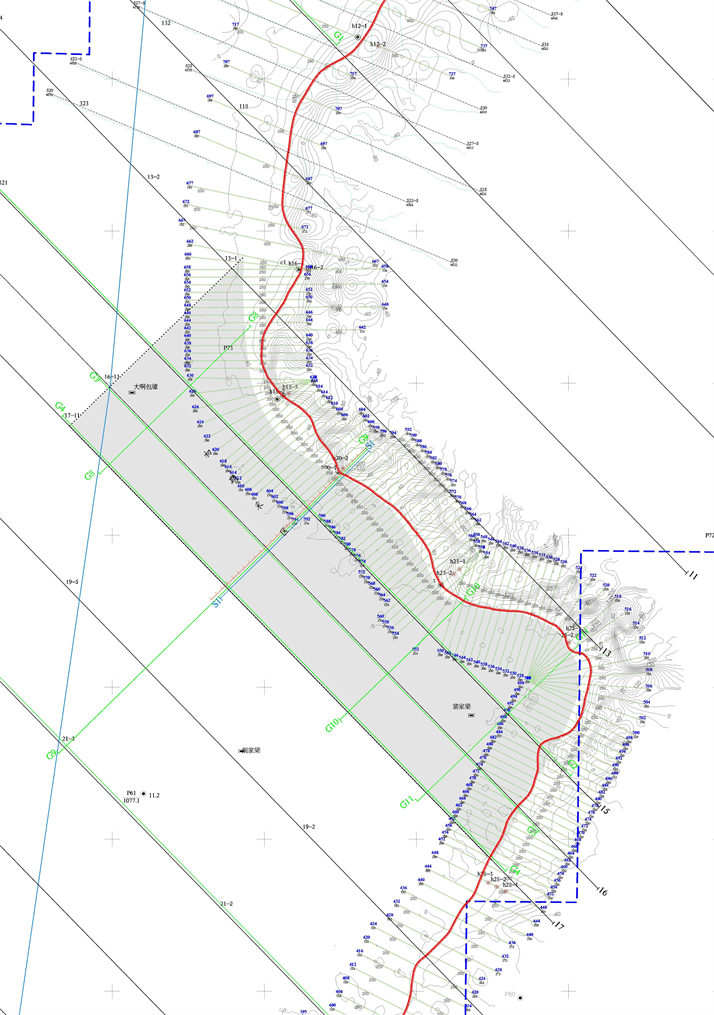# 利用高精度磁法圈定陕北地区煤层火烧边界Determining the Spontaneous Combustion Boundary of Northern Shaanxi Coal Seam Using High Precision Magnetic Method

• 全文下载: PDF(1997KB)    PP.360-367   DOI: 10.12677/AG.2019.95040
• 下载量: 244  浏览量: 342   科研立项经费支持

Based on the analysis of the spontaneous combustion process of coal seam in a mining area in northern Shaanxi Province, this paper establishes the oblique step model including normal coal seam, extinguishing zone, self-combustion variable belt and so on, and carries on the forward calculation of numerical simulation of theoretical model, synthetically analyzes and studies the measured data of magnetic method, and adopts the anomaly feature point method. The fire boundary of coal seam is accurately identified by high precision magnetic exploration, and the exploration effect is good by combining drilling data and geological data of field investigation. The research results are applied in the coalfield of northern Shaanxi province, which can provide a strong basis for the calculation of reserves, fired areas boundary division and safe production and design of the well field, and better serve the decision-making and planning of the government management department.

1. 前言

2. 理论模型设计与仿真Figure 1. Theoretical layered magnetic body model and magnetic anomaly response curve

${H}_{a}=\mathrm{sin}{i}_{s}{H}_{a}^{k}-\mathrm{cos}{i}_{s}{Z}_{a}^{k}$ (1)

${Z}_{a}=\mathrm{cos}{i}_{s}{H}_{a}^{k}+\mathrm{sin}{i}_{s}{Z}_{a}^{k}$ (2)

$\Delta T=\mathrm{sin}\left({i}_{s}^{0}+{i}_{s}\right){H}_{a}^{k}-\mathrm{cos}\left({i}_{s}^{0}+{i}_{s}\right){Z}_{a}^{k}$ (3)

$\left\{\begin{array}{c}{H}_{a}^{k}=\frac{{\mu }_{0}}{2\text{π}}{\int }_{S}\frac{{M}_{s}}{{r}^{4}}\left\{2\left(z-\zeta \right)\left(x-\xi \right)\right\}\text{d}S\\ {Z}_{a}^{k}=\frac{{\mu }_{0}}{2\text{π}}{\int }_{S}\frac{{M}_{s}}{{r}^{4}}\left\{{\left(z-\zeta \right)}^{2}-{\left(x-\xi \right)}^{2}\right\}\text{d}S\end{array}$ (4)

3. 典型实例Figure 2. Location map of the measuring areaFigure 3. Measurement curve and interpretation results of drilling position

4. 平面探测成果Figure 4. Magnetic anomaly profile floor plan and results diagramFigure 5. Magnetic anomaly contour plan and result diagram

5. 结论

  滕吉文. 强化第二深度空间金属矿产资源探查, 加速发展地球物理勘探新技术与仪器设备的研制及产业化[J]. 地球物理学进展, 2010, 25(3): 729-748.  齐朝华. 煤层火烧区的TEM响应及视电阻率分布规律研究[D]: [硕士学位论文]. 邯郸: 河北工程大学, 2012.  陈小龙, 任金礼. 高精度磁测在陕北煤田火烧区的应用[J]. 陕西地质, 2013, 31(2):76-80.  郭毅. 煤田火烧区物探勘探方法浅析[J]. 新疆有色金属, 2011, 34(4): 24-26.  蒋泽泉, 孟庆超, 王宏科. 陕西神南矿区煤炭开采保水煤柱留设分析[J]. 中国地质灾害与防治学报, 2011, 22(2): 87-91.  李涛. 陕北煤炭大规模开采含隔水层结构变异及水资源动态研究[D]: [博士学位论文]. 徐州: 中国矿业大学, 2012.  宁靖, 张建民, 宁书年. 磁导成像方法研究及在宁夏汝箕沟煤田火区探测中的应用[J]. 煤炭学报, 2001, 26(3): 66-69.  徐跃军. 浅谈煤田火烧层钻井技术[J]. 新疆有色金属, 2010, 33(6): 25, 27.  李斯昊, 张景岗. 同位素测氡法在浅煤层地质火源探测中的应用[J]. 华北科技学院学报, 2011, 8(2): 9-11.  卢国栋. 大面积煤田火区范围圈划及燃烧机理研究[D]: [博士学位论文]. 北京: 中国矿业大学, 2009.  万兆昌. 综合物探圈定多煤层自燃边界[J]. 中国煤田地质, 1996, 8(2): 38-40.  王星明, 郭栋, 马伏生. 磁法在煤田自燃区勘探中的应用[J]. 陕西煤炭, 2008, 27(4): 49-50.  张秀山. 新疆煤田火烧区特征及灭火问题探讨[J]. 中国煤田地质, 2004, 16(1): 18-21.  武建军, 蒋卫国, 刘晓晨, 等. 地下煤火探测、监测与灭火技术研究进展[J]. 煤炭学报, 2009, 35(12): 1669-1674.  邹建. 重磁交互式正反演计算研究和应用[D]: [硕士学位论文]. 成都: 成都理工大学, 2013.  吴晓瑞, 刘岩波, 高峰. 地面高精度磁测在划定煤田火烧区中的应用[J]. 中州煤炭, 2013(6): 62-64.  肖海红, 陈虎维, 李霞. 卫星遥感技术在煤田火区探测与监测中的应用研究[J]. 煤炭工程, 2009(2): 117-119.  张建星. 枣泉煤矿T-2火烧区下开采岩层移动规律研究[D]: [硕士学位论文]. 西安: 西安科技大学, 2009.  张福英, 齐福辉, 武军. 浅析东胜煤田地下煤火的探测方法[J]. 内蒙古煤炭经济, 2010(5): 65-67.